# Optimization formulation Optimization methods help us find solutions

• Slides: 12Optimization formulation • Optimization methods help us find solutions to problems where we seek to find the best of something. • This lecture is about how we formulate the problem mathematically. • In this lecture we make the assumption that we have choices and that we can attach numerical values to the ‘goodness’ of each alternative. • This is not always the case. We may have problems where the only thing we can do is compare pairs of alternatives and tell which one is better, but not by how much. • Can you think of an example?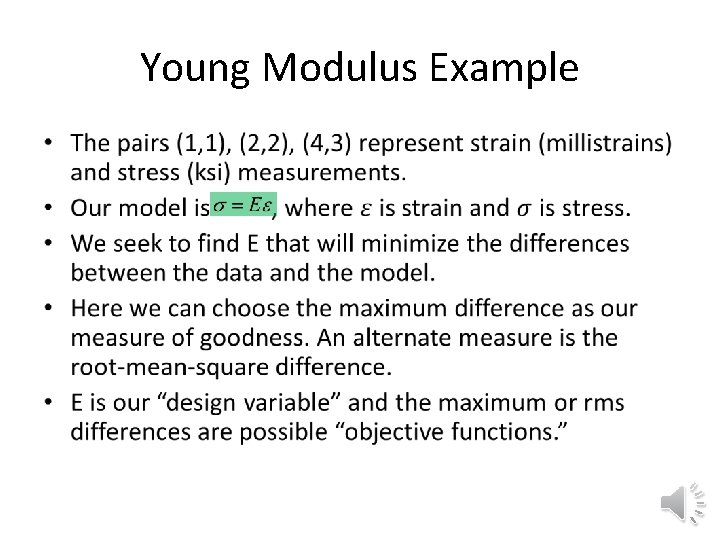Young Modulus Example •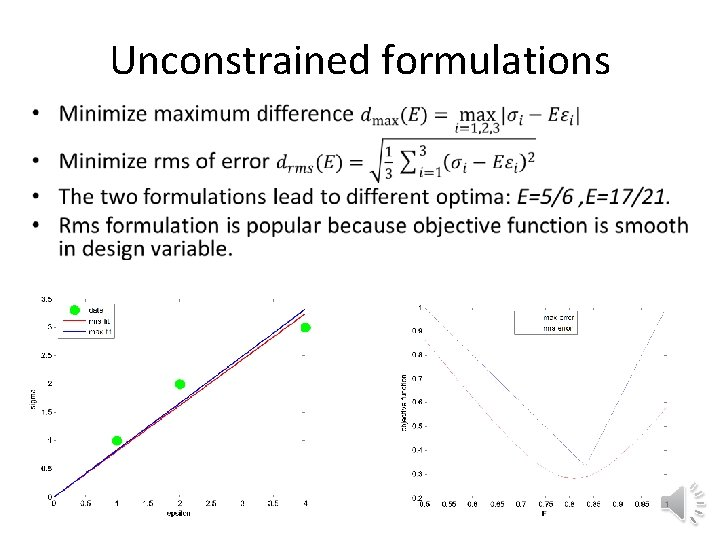Unconstrained formulations •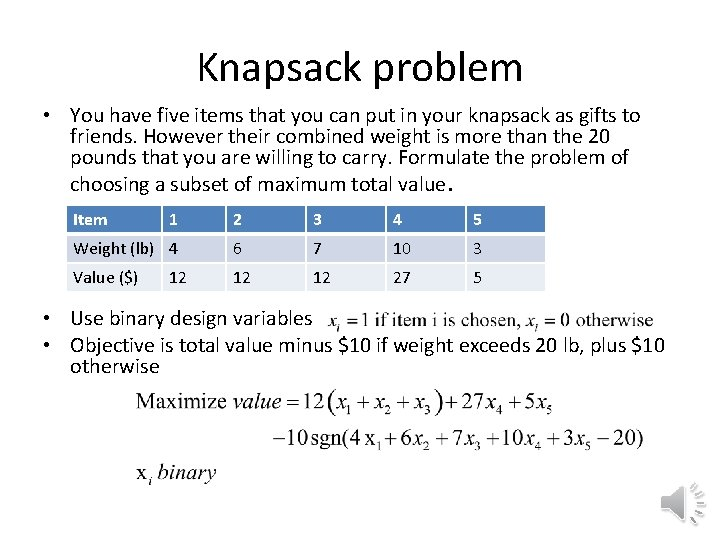Knapsack problem • You have five items that you can put in your knapsack as gifts to friends. However their combined weight is more than the 20 pounds that you are willing to carry. Formulate the problem of choosing a subset of maximum total value. Item 1 2 3 4 5 Weight (lb) 4 6 7 10 3 Value (\$) 12 12 27 5 12 • Use binary design variables • Objective is total value minus \$10 if weight exceeds 20 lb, plus \$10 otherwise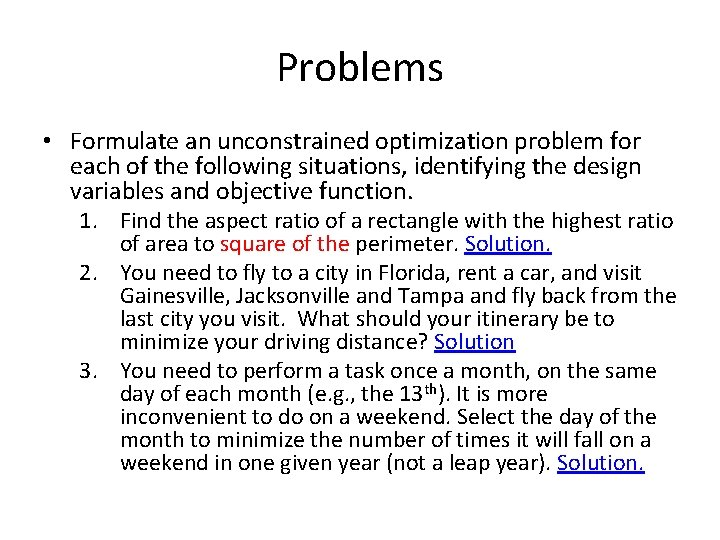Problems • Formulate an unconstrained optimization problem for each of the following situations, identifying the design variables and objective function. 1. Find the aspect ratio of a rectangle with the highest ratio of area to square of the perimeter. Solution. 2. You need to fly to a city in Florida, rent a car, and visit Gainesville, Jacksonville and Tampa and fly back from the last city you visit. What should your itinerary be to minimize your driving distance? Solution 3. You need to perform a task once a month, on the same day of each month (e. g. , the 13 th). It is more inconvenient to do on a weekend. Select the day of the month to minimize the number of times it will fall on a weekend in one given year (not a leap year). Solution.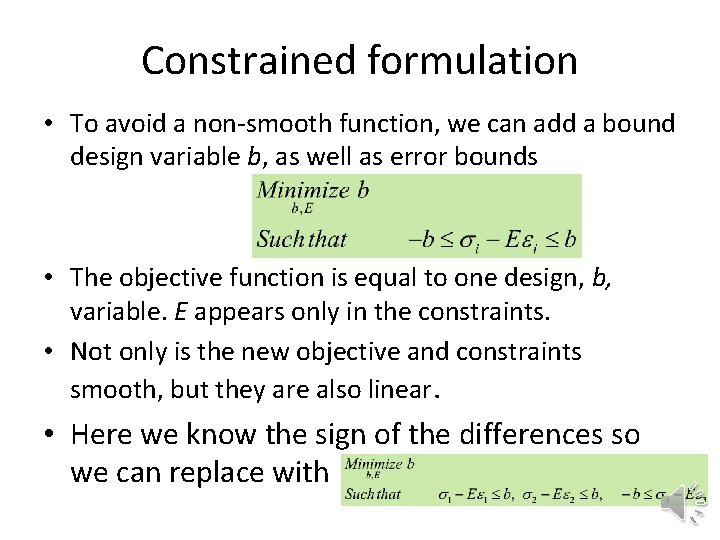Constrained formulation • To avoid a non-smooth function, we can add a bound design variable b, as well as error bounds • The objective function is equal to one design, b, variable. E appears only in the constraints. • Not only is the new objective and constraints smooth, but they are also linear. • Here we know the sign of the differences so we can replace withGraphical optimization • Recall the linear constrained optimization formulation. • With only two design variables we can plot it. • Optimum at constraint boundary intersection.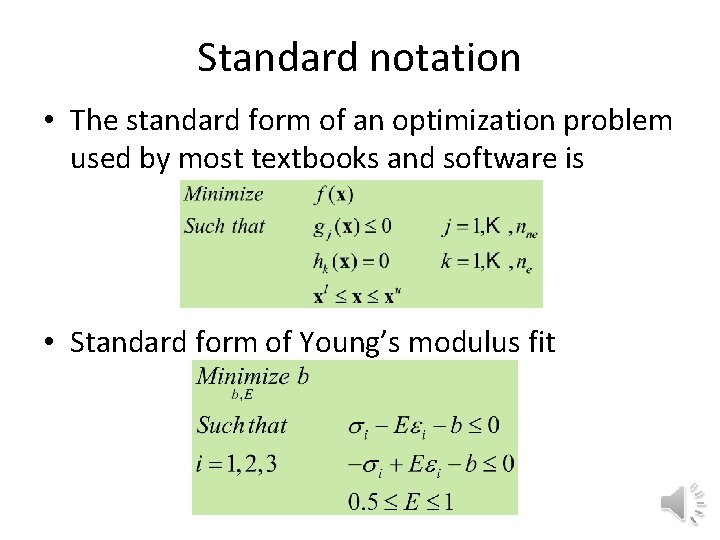Standard notation • The standard form of an optimization problem used by most textbooks and software is • Standard form of Young’s modulus fit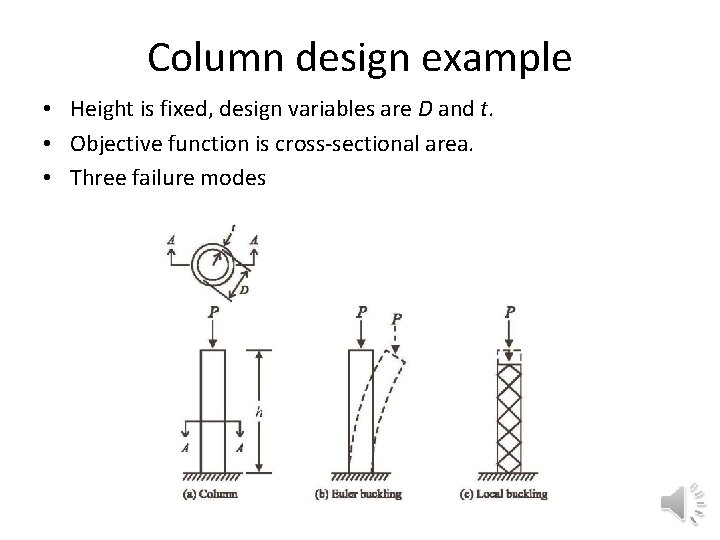Column design example • Height is fixed, design variables are D and t. • Objective function is cross-sectional area. • Three failure modes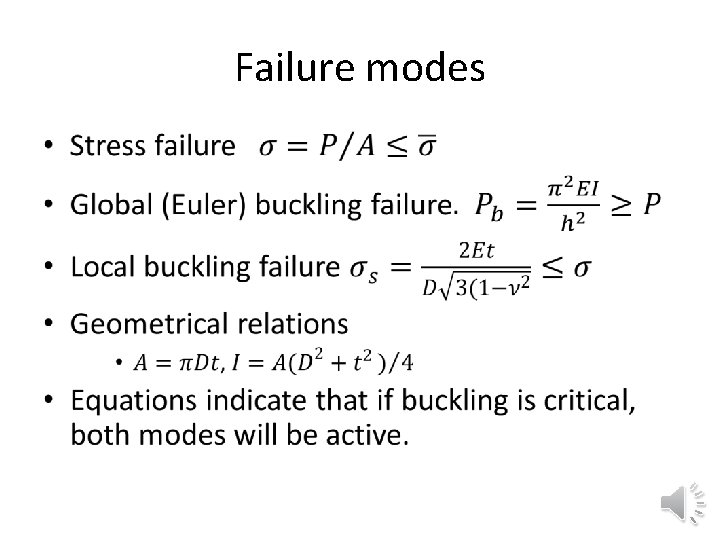Failure modes •Standard dimensionless formulation • Normalized constraints are better both numerically and for communicating degree of satisfaction.Problems • Provide two formulations in standard form for minimizing the surface area of a cylinder of a given volume when the diameter and height are the design variables. One formulation should use the volume as equality constraint, and another use it to reduce the number of design variables. Solution. • Formulate in standard normal form the problem of finding an open-top rectangle with an area of at least 50 and minimum perimeter. Solution. • You need to go from point A to point B in minimum time while maintaining a safe distance from point C. Formulate an optimization problem in standard normalized form to find the path with no more than three design variables when A=(0, 0), B=(10, 10), C=(4, 4), and the minimum safe distance is 5. Solution